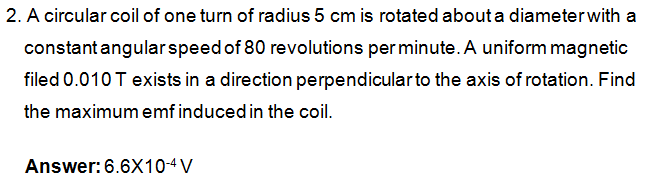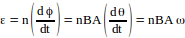## Guest8 years ago
p { margin-bottom: 0.25cm; line-height: 120%; }

From the relation.

Here

ε = emf produced in the coil

n = number of turns = 1

B = magnetic field induction = 0.010 T

A = area of the coil = π(0.05)2 = 0.00785 m2

ω = angular velocity of the coil = 80 RPM = 2π * 80/60 = 8.378 rad/sec.

Substituting all values, you get ε = 6.6 x 10-4 volts.